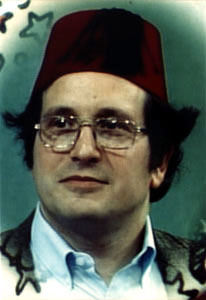# Lisp interpreter in Lisp### Introduction

Since the end of August, every saturday my colleagues at Bookmate and I are watching “Structure and Interpretation of Computer Programs” course lectures. Here’s excerpt from the course culmination - The Metacircular Evaluator.### Eval

Eval takes as arguments an expression and an environment. It classifies the expression and directs its evaluation. Eval is structured as a case analysis of the syntactic type of the expression to be evaluated. In order to keep the procedure general, we express the determination of the type of an expression abstractly, making no commitment to any particular representation for the various types of expressions. Each type of expression has a predicate that tests for it and an abstract means for selecting its parts. This abstract syntax makes it easy to see how we can change the syntax of the language by using the same evaluator, but with a different collection of syntax procedures.

Primitive expressions

• For self-evaluating expressions, such as numbers, eval returns the expression itself.
• Eval must look up variables in the environment to find their values.

Special forms

• For quoted expressions, eval returns the expression that was quoted.

• An assignment to (or a definition of) a variable must recursively call eval to compute the new value to be associated with the variable. The environment must be modified to change (or create) the binding of the variable.

• A lambda expression must be transformed into an applicable procedure by packaging together the parameters and body specified by the lambda expression with the environment of the evaluation.

• A case analysis (cond) is transformed into a nest of if expressions and then evaluated.

Combinations

• For a procedure application, eval must recursively evaluate the operator part and the operands of the combination. The resulting procedure and arguments are passed to apply, which handles the actual procedure application.
``````(define eval
(lambda (exp env)
(cond
((number? exp) exp)
((symbol? exp) (lookup exp env))
((eq? (car exp) 'quote) (cadr exp))
((eq? (car exp) 'lambda) (list 'closure (cdr exp) env))
((eq? (car exp) 'cond) (evcond (cdr exp) env))
(else
(apply (eval (car exp) env)
(evlist (cdr exp) env))))))
``````

### Apply

Apply takes two arguments, a procedure and a list of arguments to which the procedure should be applied. Apply classifies procedures into two kinds:

• it calls apply-primop to apply primitives
• it applies compound procedures by sequentially evaluating the expressions that make up the body of the procedure. The environment for the evaluation of the body of a compound procedure is constructed by extending the base environment carried by the procedure to include a frame that binds the parameters of the procedure to the arguments to which the procedure is to be applied
``````(define apply
(lambda (proc args)
(cond ((primitive? proc) (apply-primop proc args))
((eq? (car proc) 'closure)
args
(else "error"))))
``````

### Misc

``````(define evlist
(lambda (l env)
(cond ((eq? l '()) '())
(else
(cons (eval (car l) env)
(evlist (cdr l) env))))))

(define evcond
(lambda (clauses env)
(cond ((eq? clauses '()) '())
((eq? (caar clauses) 'else) (eval (cadar clauses) env))
((false? (eval (caar clauses))) (evcond (cdr clauses) env))
(else

(define bind
(lambda (vars vals env)
(cons (pair-up vars vals) env)))

(define pair-up
(lambda (vars vals)
(cond
((eq? vars '())
(cond ((eq? vals '()) '())
(else (error "Too Many Arguments"))))
((eq? vals '()) (error "Too Few Arguments"))
(else
(cons (cons (car vars) (car vals))
(pair-up (cdr vars) (vdr vals)))))))

(define lookup
(lambda (sym env)
(cond ((eq? env '()) (error "Unbound Variable"))
(else
((lambda (value-cell)
(cond ((eq? value-cell '())
(lookpu sys (cdr env)))
(else (cdr value-cell))))
(assq sym (car env)))))))

(define assq
(lambda (sym list-of-pairs)
(cond ((eq? list-of-pairs '()) '())
((eq? sym (caar list-of-pairs)) (car list-of-pairs))
(else
(assq sym (cdr list-of-pairs))))))
``````

### WizardCategories:

Updated: